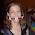## Friday, February 01, 2008

### Ed and Edd, But Not Eddy

Ed and Edd decided to build competing roller coasters. Ed built his roller coaster 40 feet high that will travel down on a straight slope. Edd built his roller coaster 40 feet high, but his travels down a curved slope (steep at the beginning, levels out at the end with no bumps).

If both cars are released at the same time (they cars are identical), which one will arrive at the bottom first?

1.curved slope will win

2.The curved slope will win because you will pick up more speed at the beginning.

3.mike - are you sure about this? I spent a lot of time considering this (this happens to be right up my alley, as you may know by now), and I think I may disagree. you do in fact get more speed at the beginning, but you have a greater distance to travel, since you have to travel the length of the line, and if the line is curved, it will be longer (i.e. the shortest distance between two points is a straight line).

Consider the following extreme example. Let's say that your "curved line" consists of a vertical drop for the full height, then a flat line going to the end, while the "straight line" coaster is just a linear slope between the start and end. We'll say that it's 45 degrees for simplicity (that is, length=height=h). If you crunch the numbers, it comes out that it takes slightly longer to get to the end of the "curved" coaster, unless I made a mistake.

"Straight Line"
distance to travel = d = h*sqrt(2)
acceleration = a = g cos(theta) = g/sqrt(2)
d = h*sqrt(2) = (1/2)*a*t^2
t = 2*sqrt(h/g)

"Curved Line"
First Leg:
distance = d = h
acceleration = a = g
d = h = 1/2*a*t1^2
t1 = sqrt(2*h/g)
final velocity = v = sqrt(2*g*h)

Second Leg:
distance = d = h
acceleration = a = 0
d = v*t2
t2 = d/v = h/sqrt(2*g*h) = sqrt(h/(2*g))

t = t1+t2 = (sqrt(2)+sqrt(1/2))*sqrt(h/g)

since (sqrt(2)+sqrt(1/2))>2, the "curved" scenario takes longer, by my figuring. Anyway, it seems like this would hold true for all similar scenarios if it held true for this case, but I'm not sure about it.

I bet this was a lot more than you bargained for on this question...sorry.

4.As usual for me, I'm not sure. It's going to take me some time to figure this one out.

At first glance, I would think it would matter how far you are dropping. Off the top of my head (I haven't worked my way through your math, yet) I can definitely see there would be a tipping point where the straight line would catch up to the curved line, but for a short drop, the curved line (I would think) would win.

And, if there is a tipping point, I wonder what that would be.

And, that all supposes that I'm right about the quick pick-up of speed.

Let me work through your math and see what I can see.

5.Alright, after trying my hand at some algebra, I think I came up with time on a curved track is (sqrt(2) + sqrt(2))*sqrt(h/g) which is bigger than time on a straight track 2*sqrt(h/g).

If either of us is doing the math correctly, the straight line wins.

6.it's ok. I'm going to actually look at some Hamiltonian / Lagrangian techniques and see if I can solve this definitively with calculus of variations. you've piqued my curiosity. I'll get back to you.

7.Mike,
I crunched the numbers on one that slopes just a little more sharply than a straight line, then levels off at the bottom, and it is indeed faster. If you slope just a little more at the first, it is faster, but the extreme case I gave is slower, which means there is some maximization curve which will do it in the fastest time. Anyway, good question, even if you didn't realize it at the time ;-)

8.http://en.wikipedia.org/wiki/Brachistochrone

9.Wow, that was totally not obvious to me. Very interesting though.

I really wish I could say I knew it all along, but I'm guessing everyone here would know better by now!

Leave your answer or, if you want to post a question of your own, send me an e-mail. Look in the about section to find my e-mail address. If it's new, I'll post it soon.

Please don't leave spam or 'Awesome blog, come visit mine' messages. I'll delete them soon after.

Enter your Email and join hundreds of others who get their Question of the Day sent right to their mailbox

The Lamplight Manor Puzz 3-D